Normal view

# Bayesian data analysis / Andrew Gelman, John B. Carlin, Hal S. Stern, David B. Dunson, Aki Vehtari, Donald B. Rubin.

Material type:TextLanguage: English Edition: Third editionDescription: pages cmISBN: 9781439840955 (hardback); 1439840954DDC classification: 519.542 LOC classification: QA279.5 | .G45 2014Other classification: MAT029000 Online resources: Cover image | WorldCat Details
Contents:
Table of contents FUNDAMENTALS OF BAYESIAN INFERENCE Probability and Inference Single-Parameter Models Introduction to Multiparameter Models Asymptotics and Connections to Non-Bayesian Approaches Hierarchical Models FUNDAMENTALS OF BAYESIAN DATA ANALYSIS Model Checking Evaluating, Comparing, and Expanding Models Modeling Accounting for Data Collection Decision Analysis ADVANCED COMPUTATION Introduction to Bayesian Computation Basics of Markov Chain Simulation Computationally Efficient Markov Chain Simulation Modal and Distributional Approximations REGRESSION MODELS Introduction to Regression Models Hierarchical Linear Models Generalized Linear Models Models for Robust Inference Models for Missing Data NONLINEAR AND NONPARAMETRIC MODELS Parametric Nonlinear Models Basic Function Models Gaussian Process Models Finite Mixture Models Dirichlet Process Models APPENDICES A: Standard Probability Distributions B: Outline of Proofs of Asymptotic Theorems C: Computation in R and Stan Bibliographic Notes and Exercises appear at the end of each chapter.
Summary: "Preface This book is intended to have three roles and to serve three associated audiences: an introductory text on Bayesian inference starting from first principles, a graduate text on effective current approaches to Bayesian modeling and computation in statistics and related fields, and a handbook of Bayesian methods in applied statistics for general users of and researchers in applied statistics. Although introductory in its early sections, the book is definitely not elementary in the sense of a first text in statistics. The mathematics used in our book is basic probability and statistics, elementary calculus, and linear algebra. A review of probability notation is given in Chapter 1 along with a more detailed list of topics assumed to have been studied. The practical orientation of the book means that the reader's previous experience in probability, statistics, and linear algebra should ideally have included strong computational components. To write an introductory text alone would leave many readers with only a taste of the conceptual elements but no guidance for venturing into genuine practical applications, beyond those where Bayesian methods agree essentially with standard non-Bayesian analyses. On the other hand, we feel it would be a mistake to present the advanced methods without first introducing the basic concepts from our data-analytic perspective. Furthermore, due to the nature of applied statistics, a text on current Bayesian methodology would be incomplete without a variety of worked examples drawn from real applications. To avoid cluttering the main narrative, there are bibliographic notes at the end of each chapter and references at the end of the book"--
Tags from this library: No tags from this library for this title.Average rating: 0.0 (0 votes)
Item type Current location Collection Call number Copy number Status Date due Barcode Item holdsText
Reserve Section
Non-fiction 519.542 BAY 2014 (Browse shelf) C-1 Not For Loan 25719Text
Reserve Section
Non-fiction 519.542 BAY 2014 (Browse shelf) C-2 Not For Loan 26045
Total holds: 0
##### Browsing EWU Library shelves, Shelving location: Reserve Section Close shelf browser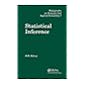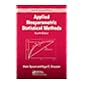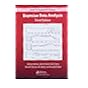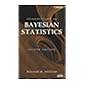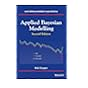519.54 SIS 2003 Statistical inference / 519.54 SPA 2007 Applied nonparametric statistical methods / 519.542 BAY 2014 Bayesian data analysis / 519.542 BAY 2014 Bayesian data analysis / 519.542 BOI 2007 Introduction to Bayesian statistics / 519.542 COA 2014 Applied Bayesian modelling / 519.542 COA 2014 Applied Bayesian modelling /

Includes bibliographical references and indexes.

Table of contents FUNDAMENTALS OF BAYESIAN INFERENCE Probability and Inference Single-Parameter Models Introduction to Multiparameter Models Asymptotics and Connections to Non-Bayesian Approaches Hierarchical Models FUNDAMENTALS OF BAYESIAN DATA ANALYSIS Model Checking Evaluating, Comparing, and Expanding Models Modeling Accounting for Data Collection Decision Analysis ADVANCED COMPUTATION Introduction to Bayesian Computation Basics of Markov Chain Simulation Computationally Efficient Markov Chain Simulation Modal and Distributional Approximations REGRESSION MODELS Introduction to Regression Models Hierarchical Linear Models Generalized Linear Models Models for Robust Inference Models for Missing Data NONLINEAR AND NONPARAMETRIC MODELS Parametric Nonlinear Models Basic Function Models Gaussian Process Models Finite Mixture Models Dirichlet Process Models APPENDICES A: Standard Probability Distributions B: Outline of Proofs of Asymptotic Theorems C: Computation in R and Stan Bibliographic Notes and Exercises appear at the end of each chapter.

"Preface This book is intended to have three roles and to serve three associated audiences: an introductory text on Bayesian inference starting from first principles, a graduate text on effective current approaches to Bayesian modeling and computation in statistics and related fields, and a handbook of Bayesian methods in applied statistics for general users of and researchers in applied statistics. Although introductory in its early sections, the book is definitely not elementary in the sense of a first text in statistics. The mathematics used in our book is basic probability and statistics, elementary calculus, and linear algebra. A review of probability notation is given in Chapter 1 along with a more detailed list of topics assumed to have been studied. The practical orientation of the book means that the reader's previous experience in probability, statistics, and linear algebra should ideally have included strong computational components. To write an introductory text alone would leave many readers with only a taste of the conceptual elements but no guidance for venturing into genuine practical applications, beyond those where Bayesian methods agree essentially with standard non-Bayesian analyses. On the other hand, we feel it would be a mistake to present the advanced methods without first introducing the basic concepts from our data-analytic perspective. Furthermore, due to the nature of applied statistics, a text on current Bayesian methodology would be incomplete without a variety of worked examples drawn from real applications. To avoid cluttering the main narrative, there are bibliographic notes at the end of each chapter and references at the end of the book"--

Applied Statistics

There are no comments for this item.